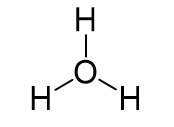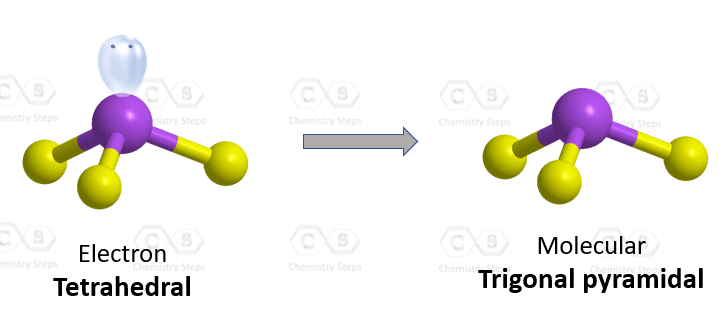## Examples

The central atom is the oxygen, so we can draw a preliminary skeletal structure:The total number of electrons is 6 + 3 – 1 = 8, out of which, 6 are used to make three covalent bonds, and one goes to the oxygen as a lone pair. The oxygen is positively charged since it only has one lone pair of electrons:There are 3 atoms and one lone pair on the central atom, therefore, the electron geometry is tetrahedral, and the molecular geometry is trigonal pyramidal.Steric number 4 corresponds to sp3-hybridization where the idealized bond angles are 109.5o. Because the groups on the central atom are not identical, the bond angles differ from the idealized values.

Check Also